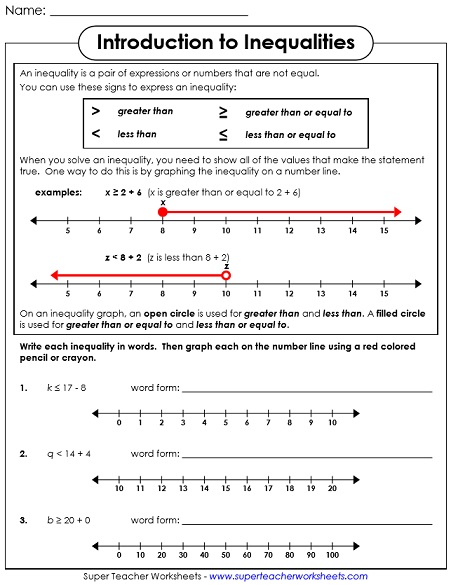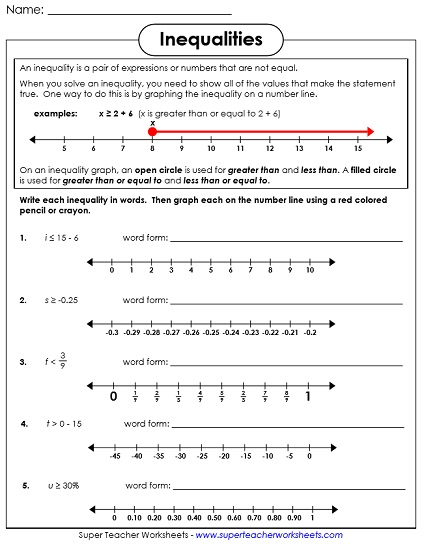# Inequalities with One Step

Graph, write, and solve one-step inequalities with these basic algebra worksheets. On these problems, students need to isolate the variable using only a single step. Then they graph the inequality.## Basic Level(Whole Numbers Only)

On this worksheet, students will find and graph solutions to basic, one-step single variable inequalities. All problems have only positive, whole numbers.
Students graph the basic, one-step inequalities. All inequalities on this worksheet have addition or subtraction on the side of the inequality as the variable.
A set of one-step, basic inequalities for students to graph on a number line. All the inequalities feature multiplication or division on the same side of the inequality as the variable.
Solve the inequality and circle the numbers that are in the solution set. This version includes only whole numbers.
Rewrite each phrase as an inequality in standard algebraic form. (example: The product of 6 and x is greater than or equal to 48.)

## Intermediate Level(Includes Neg. Numbers & Decimals)

Students must find and graph solutions to one-step single variable inequalities by isolating the variable. Uses negative numbers, fractions, decimals, and operators.
Intermediate, one-step inequalities are graphed on a number line. This worksheet includes only addition or subtraction on the same side of the inequality as the variable. Negative numbers, decimals, and fractions are included
A page of one-step intermediate-level inequalities with a multiplication or division fact on the same side of the inequality as the variable. The multiplication and division include decimals, fractions, and negative numbers.
Solve each inequality and select the answers that are in the solution set. This version includes fractions and decimals.
Rewrite each inequality in standard form. (example: The sum of -7.8 and x is less than 42.)
More Inequality Worksheets

This is our index page that shows all categories for our inequality worksheets.

## Sample Images of Our Printable WorksheetsMy Account
Site Information